Individual-based model to enrich an aggregate model

Raphaël Duboz*  *, Frédéric Amblard**, Eric Ramat*, Guillaume Deffuant**, Philippe Preux*

 *Laboratoire d'Informatique du Littoral (LIL) Maison de la Recherche Blaise Pascal 50, rue Ferdinand Buisson - BP 719 62228 Calais Cedex France **Laboratoire d'Ingénierie pour les Systèmes Complexes (LISC) Cemagref 24, avenue des Landais BP 50085 63172 Aubière Cedex France

*

tel: +33 321 465 679

Abstract: We propose to use individual-based models as virtual laboratories to identify parameters of aggregate models. We illustrate this approach with a simple example: the diffusion of particles activated by a Brownian movement. We thus have an aggregated numerical model, as well as an individual-based model of this phenomenon. We consider various alternatives of this example, which lead to different use of the virtual laboratory. We consider in particular the coupling between the two models in which the aggregate model asks for calculations of parameters to the individual-based model. We evoke finally the case of scale shifts in the models and the way it can be managed by this type of approach.

Key words: coupling, multi-formalism, differential equations, individual-based models.

# 1         Introduction

To describe a social,    a physical or a biological system, the differential equations are the fundamental tool of the scientists. This approach tends to describe a system made up of many elements by aggregate variables (densities of populations for instance). This approach is interested in the dynamics of these variables. It follows a very compact and very general representation of the evolution of the system. However, these models utilize aggregate parameters whose identification can prove to be delicate.

The individual-based models on the contrary explicitly represent the whole of the system’s elements, like their interactions. They have been used more and more largely in particular in ecology, for several years (Grimm, 1999) but also to model social systems (Epstein and Axtell, 1996). There too, the differential equations are settings in general to express the behavior of the elements of the system. However, these models find a natural expression in recent work in computer sciences, like the object-oriented programming, then the multi-agents systems (Ferber, 1995). Also, the representations of individual dynamics can sometimes deviate from a pure differential formalism. The individual-based models considered in this article are not necessarily multi-agents models, if we adopt the strict definition of Wooldridge and Jennings (Wooldridge and Jennings, 1995). We thus use the term individual-based since modeling is focused on the entities composing the system, in opposition to an approach considering of the aggregate variables representing the whole of the entities.

In fact, these two models can be seen like representations of the same system on two different levels of abstraction. In this paper, we try to show the interest to develop the two levels of abstraction together. Our principal argument is that these two levels can be shouldered mutually, i.e. to be used one the other. One can also quote several examples of practical applications in which a traditional numerical model cohabits with an individual-based model (Hill and Coquillard, forest fires). In these applications, the individual-based model is used to account for the front of propagation of the phenomenon, thanks to its flexibility in use. One can thus find a certain similarity with our own step. However, in our proposal, the individual-based model and the aggregate model relate to the same phenomenon, which is not completely the case in the preceding examples (because the individual-based model is used only for a part of the problem). We are closer to idea of decreasing abstraction from Lindenberg (Lindenberg, 1992), which possibly recommends the development of increasingly precise models, whose comprehension is nourished of that of the models of broader granularity, in different formalisms. The concept of multi-modeling (Fishwick, 1995) is also related with our step. One finds this idea there to describe a phenomenon using several models on different levels of abstraction or refinement.

We are distinguished from this work by our proposal for a particular type of co-operation between the two levels of abstraction: the use of the individual-based model like a virtual laboratory, allowing to make experiments and measurements, in order to identify parameters of the aggregate model.

To illustrate this idea concretely, we consider a simple example: diffusion in a fluid of particles activated by a Brownian movement. The aggregate model is reduced to the equation of evolution of the particles’ concentration in space. The individual-based model represents the whole of the particles, and their random movements. We consider various alternatives of this phenomenon, in which the aggregate model to identify certain parameters can call the individual-based model dynamically. We show that these operations can in certain cases resort to scales shifts. Lastly, we propose a discussion on the interest of such couplings compared to an individual-based model only, or the interest to carry out such a coupling on-line, compared to the preliminary preparation of the measures within the virtual laboratory.

# 2         Aggregate and individual-based models of particles diffusion

The diffusion of particles is a particularly simple example of phenomenon for which microscopic physical description is connected mathematically to the macroscopic description of the system by statistical mechanics. It is thus easy to work out an aggregate model and an individual-based model of this phenomenon, and to establish a link between them. This phenomenon thus appeared at the same time sufficiently simple to us to be easy to expose, and sufficiently rich to illustrate our step.

## 2.1       Aggregate model

The study of the diffusion phenomenon by the kinetic theory established the equation of Fick, aggregate model of Brownian diffusion (Fick, 1855). One is interested here only in the component along the x-axis (x):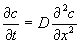(Equation 1)

Where c is the concentration in particles, D the diffusion coefficient and x the axis on which takes place the diffusion.

Two methods exist to solve a differential equation. The first consists in integrating the equation analytically, the second passes by a numerical resolution using a suitable diagram of integration.

When the initial concentration and the limits of space are known, it is possible analytically to calculate the solution of the Fick’s equation: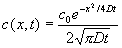(Equation 2)

Where c0 is the initial concentration, c the concentration, D the diffusion coefficient.

The calculation of this solution gives a bell-shaped curve (Gaussian) representing the concentration of a product diffusing along the x-axis at a particular time t (cf. curve in dotted lines of figure 2).

However, if it is supposed that the diffusion coefficient is variable in space, the analytical solution is not always calculable. It is then necessary to use a numerical resolution of the equation with variable coefficient and constant step (equations 3) (Dautray and Lions, 1984), by discretising space and time.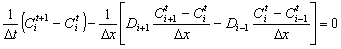(Equation 3)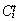is the concentration in particles with the space step i and the time step t, one supposes that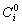is given.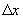the space step.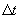the time step.

Di  the diffusion coefficient at the space step i

## 2.2       Corresponding individual-based model

The individual-based model simulating the phenomenon of diffusion is particularly simple initially, but we will see thereafter that this one can be complexified to answer more precise problems. We suppose that the particles evolve/move in a cube of three dimensions (x, y, z). We suppose for example that the particles are distributed initially at random on the plan defined by x=0, separating the cube in two halves of the same volume. Simulation consists in giving a direction and a random speed (between 0 and vmax) to each particle, for each iteration. The boundary condition of the cube is the impossibility for any particle of leaving; one thus withdraws at random a direction and a speed for any particle that leaves the cube. This model appears mainly stochastic. It is thus necessary to simulate a huge number of particles so that the observation of the behavior of the model is not a particular event but the reflection of an average behavior of the whole of the particles. At every moment, this model gives the position of all the particles. It thus makes it possible to calculate the concentration in particles in every part of space, but also possibly of other indicators.

# 3         Virtual laboratory for the determination of parameters

The aggregate model can thus give us an estimate of the diffusion parameter D of the aggregate model, corresponding to a maximum speed of the particles.

For that, one regards the individual-based model as a virtual laboratory, on which one practices experiments and measurements (Legay, 1997; Grimm, 1999). Thus, on the basis of a random distribution of the particles in the plan x=0, one measures at every moment t, the average standard deviation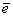of the whole of the particles, i.e. the square root of the sum of distances squares between the particles and the origin of the x-axis divided by the number of particles.

One can deduce the diffusion coefficient D from it in an experimental way, using the relation: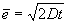then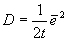(equation 4)

Where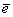is the average standard deviation, D the coefficient of diffusion and t time.

Figure 1 shows the evolution of the quantity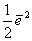according to time. It is by calculating the slope of this line that we can obtain the diffusion coefficient D.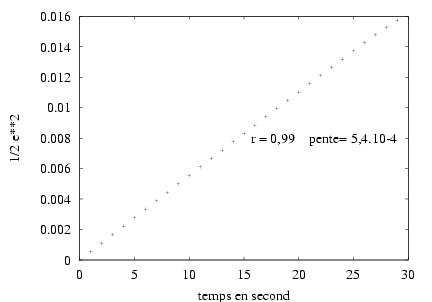Figure 1:according to time. The slope of this line is the diffusion coefficient D.

Then, one can check that the microscopic model of the particles has the same behavior as the macroscopic equation of Fick. Figure 2 makes it possible to compare the two models.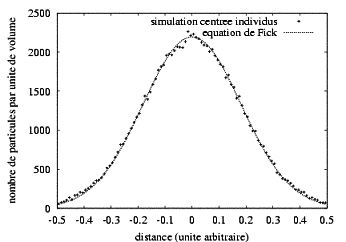Figure 2: Individual-based simulation for 105 particles (cross) and the equation of Fick (dotted line) with t=30s. The simulation and the theoretical curve correspond almost perfectly. One regards the two models as equivalents by their execution trace.

Figure 2 shows us the results of a simulation of the diffusion carried out with 105 particles for a 30 seconds simulated duration in a cube of 1cm3. Speed is determined at random from a uniform law between 0 and vmax=0.1cm.s-1. Equation 5 gives us the diffusion coefficient D = 5.10-4 s-2. Individual-based simulation is adjusted very well with the equation of Fick. A test of the c2 indicates that the two distributions are not significantly different: p(c2 =  24,76) > 0,5 with 30 degrees of freedom. This result is not surprising since we do nothing but reproduce the experiment carried out in laboratory by physicist J.B. Perrin and his students to measure the diffusion coefficient (Perrin, 1997). The first use that we thus make of multi-modeling within this framework thus allows the determination of particular values of parameters or functions of these parameters. The individual-based model is then regarded as a virtual laboratory in which we conduce experiments on the system to determine parameters of the aggregate model, here D. That we can thereafter integrate into the differential equation (cf. equation 1) for an analytical resolution.

# 4         Coupling of the two models

In the preceding paragraph and the former work applied to marine ecology (Duboz and Al, 2001), we showed that it was possible to parameterize an analytical equation using an individual-based model. We will show here that it is also possible to parameterize a numerical scheme during its resolution. In the majority of the cases, we do not have an expression of D according to vmax which could be directly used in equation 2. We thus simulate the phenomenon using the individual-based model to determine, for each iteration, and for each section of the cube the value of Di. Which we reintroduce in the equation of the numerical model for then determining by resolution of the numerical model the values of the various concentrations in each section of space (cf Figure 3). To identify each Di, we use the method presented at the preceding paragraph, by using an individual-based model on 31 individuals only to limit the calculation time. We checked that the results are equivalent to those obtained by simulating 105 individuals.

Even if the conditions of simulation remain identical, the great stochasticity of the individual-based model leads to variable values of Di in a small interval. For the calculation of such a numerical scheme, it is thus significant to know the field of variation of Di in order to observe the conditions of stability.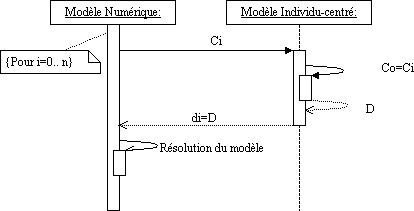Figure 3: UML Sequence diagram for the illustration of the bi-directional coupling between numerical model and individual-based model, for each section of space i, the digital model will launch an individual-based model initialized with the corresponding concentration to determine the coefficient of diffusion in the section.

Figure 3 illustrates the coupling of the two models. Such a coupling makes it possible to simulate the space effects of the variation of the diffusion coefficient on the system dynamics. We will illustrate this method by considering that the concentration of the particles has an effect on their speed, for example by considering an escape reaction of the ones compared to the others. This reaction to the concentration can be modeled by a Monod type equation which makes increase speed according to the concentration until a fixed maximum (equation 5).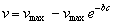(equation 5)

Where v is the speed of the particles, vmax the maximum speed and c the concentration.

The model is the same one as previously with for the expression the particles speed vmax = 0.5 cm.s-1 and b = 10-3. We obtain the results illustrated by figure 4. The difference with the preceding case is that the individual-based model at every moment needs the value of the local concentration, which is given by the aggregate model. With such an assumption of modeling, figure 4 shows that the particles do not reach the edge of the cube for the same duration of simulation and that the bell form of the equation of Fick is not preserved for the small concentrations. There is no question here of making a precise study of this phenomenon but of illustrating the coupling like a method of possible investigation.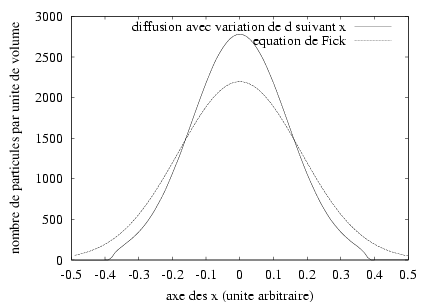# 5         Discussion

In the two examples given previously (parameterization and coupling), we simulated the same duration in the same space for the same concentration. However, the same methods can be applied to different scales. In our toy example, the scaling does not modify a priori dynamic thus the method is not of interest in this precise case. We describe however how a scaling could take place, at only illustrative ends. Let us consider the individual-based model simulating the diffusion of a small number of particles in a small volume and, by using a bi-directional coupling and the same assumptions that previously, one provides to the integration scheme the value of the diffusion coefficient to each time step, this last being higher than the time simulated by the individual-based model and for each space step, larger than the cube simulated by the individual-based model. Using a simple operator who makes shift the unit of the diffusion coefficient to give it to the integration scheme, we simulate a scale transfer.

This type of coupling can be particularly interesting for the simulation of the animals migrations for example, on a regional scale modeled using differential equation, knowing that the individual behavior and the reactions to the environment strongly influence the dynamics of the whole of the herd. The individual-based model of "water balls" proposed by Servat (Servat 2000), could be the subject of a coupling with various aggregate models (different according to the water quantity) usefully inspired of this approach. One can suppose that it is also the case of many other applications. Since the methods of Monte-Carlo, it is known that simulations having recourse to the pseudo-random numbers can for example be used for the calculation of integrals presenting limits of integration which are not easy to express analytically (Press and Al, 1994). Simulation assists "here" mathematics, when those reach their operational calculative limits. In the same way the methods of numerical integration, although resulting from theorems and axioms shown formally, were born from the need to solve numerically, i.e. by simulating them, the generally analytically insoluble systems of differential equations. Our approach is in the same line, by adding the idea of virtual experimentation on the individuals-centered model.

We think that this approach makes it possible in certain cases as well as possible to use the advantages of the two types of modeling, and to obtain a real appreciation compared to the use of only one of the models. Indeed, the aggregate model makes it possible to obtain a compact and easily usable representation of the whole of the model’s dynamics. The individual-based model makes it possible to soften the use of the aggregate model by identifying its parameters locally. One can in addition put the question of the effectiveness of the on-line coupling. It is clear that in certain cases it can be more advantageous to draw up a priori a protocol of tests in the virtual laboratory and to draw from it the interesting values for the aggregate model. It is the case in our example if the whole of the reachable values of vmax are known a priori. Indeed, it is then enough to establish experiments for various values of vmax in this interval, in order to obtain by interpolation the function which gives D according to vmax. This function is then directly used in the aggregate model. It is a generic method to many other models.

# 6         References

Dautray R. et Lions J.L., 1984. Analyse mathématique et calcul numérique pour les sciences et les techniques. Evolution : numérique, transport, vol 9, INSTN CEA, 1984.

Duboz R., Ramat E. et Preux P., “Towards a coupling of continuous and discrete formalism in ecological modelling: influences of the choice of algorithms on results”, in proceedings of the ESS’01 conference, Marseille (France), p.481-487, 2001

Epstein, J. and Axtell, R., 1996. Growing artificial societies, Social Science from the bottom-up, MIT Press.

Ferber, J., 1995. Les systèmes Multi-agents, vers une intelligence collective. Inter-editions, 1995.

Fick, A., 1855. „Über diffusion“, Annalen der Physik und Chemie, 94, p.59-86. 1855.

Fishwick P., 1995. Simulation Model design and Execution. Building Digital Worlds. Prentice Hall, 1995.

Forrest S., “Emergent computation : self organizing, collective, and cooperative phenomena in natural and artificial networks, Introduction to the proceedings of the ninth annual CNLS conference”, Physica D 42, p1-11, 1990.

Grimm, V., 1999. “Ten years of individual-based modelling in ecology: what we have learned and what could we learn in the future ?”, Ecological Modelling, 115, p.129-148, 1999.

Legay, J.-M., 1997. L’expérience et le modèle, Un discours sur la méthode. INRA éditions, 1997.

Lindenberg, S., 1992. “The method of decreasing abstraction.” In Rational choice theory: advocacy and critique. T.Fararo (ed.), vol.7, Sage publications, p.3-20, 1992.

Perrin, J.-B., 1997. L’ordre du chaos. Bibliothèque Pour La Science, Pour La Science eds, 1997.

Press W. H., Teukolsky S. A., Vetterling W. T., Flannery B. P., Numerical Recipies in C, (FORTRAN, PASCAL), The art of Scientific Computing, 2nd Ed, Cambridge University Press, 1994.

Servat, D. Modélisation de dynamique de flux par agents. Application aux processus de ruissellement, infiltration et érosion. PhD Thesis Paris VI, 162p, 2000.

Wooldridge, M. and Jennings, N., 1995. “Intelligent Agents: Theory and Practice.”, The Knowledge Engineering Review, 10(2), pp. 115-152, 1995.